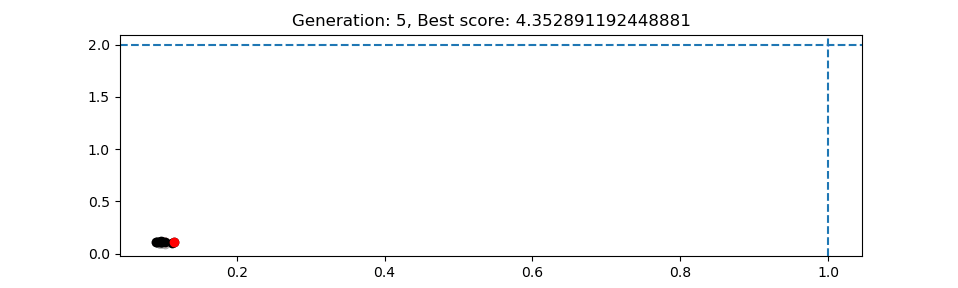# Optimisation¶

This is an experimental feature which is at an early stage of development. The aim is to enable equilibria to be automatically optimised. This has the following components:

1. Measures, a quantity which measures how “good” a solution is. Typically the aim is to minimise this quantity, so I suppose it’s really a measure of how bad the solution is.
2. Controls, quantities which can be changed. These could be machine parameters such as coil locations, constraints like X-point location, or plasma profiles such as poloidal beta or plasma current.
3. An algorithm which modifies the controls and finds the best equilibrium according to the measure it’s given. At the moment the method used is Differential Evolution.

## Differential Evolution¶

Differential Evolution is a type of stochastic search, similar to Genetic Algorithms, generally well suited to problems involving continuously varying parameters.

The implementation of the algorithm is in freegs.optimiser. It is generic, in that it operates on objects but does not need to know any details of what those objects are. To modify objects a list of `controls` are passed to the optimiser, each of which can set and get a value. To score each object a `measure` function is needed, which takes an object as input and returns a value. The optimiser works to minimise this value.

An example which uses the optimisation method is in the `freegs` directory. This optimises a quadratic in 2D rather than tokamak equilibria. 100 generations are run, with 10 solutions (sometimes called agents) in each generation. Run this example with the command:

```python test_optimiser.py
```

This should produce the figure below. The red point is the best solution at each generation; black points are the other points in that generation. Faded colors (light red, grey) are used to show previous generations. It can be seen that the points are clustered around the starting solution, as the agents spread out, and then around the solution as the agents converge to the minimum.## Optimising tokamak equilibria¶

The code specific to FreeGS optimisation is in freegs.optimise. This includes controls which modify aspects of the tokamak or equilibrium:

Control Description
CoilRadius(name [, min, max]) Modify coil radius, given name and optional limits
CoilHeight(name [, min, max]) Modify coil height

Measures which can be used by themselves or combined to specify the quantities which should be optimised:

Measure function Description
max_abs_coil_current Maximum current in any coil circuit
max_coil_force Maximum force on any coil
no_wall_intersection Prevent intersections of wall and LCFS

Each measure function takes an Equilibrium as input, and returns a value. These can be combined in a weighted sum using `optimise.weighted_sum`, or by passing your own function to `optimise`.

The example 11-optimise-coils.py uses the following code to reduce the maximum force on the coils, while avoiding wall intersections:

```from freegs import optimise as opt

best_eq = opt.optimise(eq,  # Starting equilibrium
# List of controls
The monitor should be a callable (here it is an object of class `PlotMonitor`), which is called after each generation. This is used to update a plot showing the best solution in each generation, and save the figure to file.